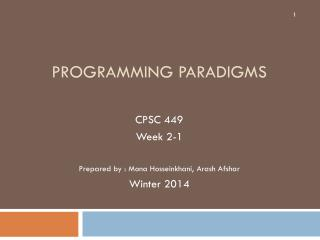DownloadDownload PresentationTélécharger la présentation- - - - - - - - - - - - - - - - - - - - - - - - - - - E N D - - - - - - - - - - - - - - - - - - - - - - - - - - -
##### Presentation Transcript

1. Programming Paradigms CPSC 449 Week 2-1 Prepared by : Mona Hosseinkhani, ArashAfshar Winter 2014

2. Some Excercises • Load the file FirstScript.hs and evaluate the following expressions: • square size • let d = double 2 • 23 - double (3+1) • 23 - double 3+1 • Use :type to tell the type of each of the above expressions. • Edit the file FirstScript.hs to include the following definitions • The function should double its input and square the result of that. • The function should square its input and double the result of that.

3. Integer type and some of its operations • Arithmetic operators • +, *, ^, - ,… • div, mod, abs, negate • Relational Operators • >, >=, <, <=, /=, == • fromInteger :: Integer -> Int • toInteger :: Int -> Integer

4. Char and String types and some of their operations • fromEnum :: Char -> Int • toEnum :: Int -> Char • putStr :: String -> IO () • putStrLn:: String -> IO () • ++ : concatenates two Strings • show : Converts from a value to a String • Show (2+3) • read : Converts a String to a value • read “True”

5. Redefining Prelude functions • The “max” function is defined both in the Prelude.hs and Chapter3.hs • Max :: Integer -> Integer -> Integer • Ambiguous occurrence `max’It could refer to either `Chapter3.max’,defined at Chapter3.hs:70:1or `Prelude.max’,imported from `Prelude' at Chapter3.hs 11:8-15 (and originally defined in `GHC.Classes’) • Import Prelude hiding (max)

6. Syntax • A function definition ends by the first piece of text which lies at the same indentation or to the left of the start of the definition. (offside rule) e.g. mystery x = x * x +x +2 next y =… • Incorrect syntax: • Funny x = x+1 • In Haskell end symbol is ‘;’. Use it when you want to write more than one definition on a single line.

7. Summary • Installing Haskell platform and downloading the textbook codes. • Fire up GHCi (GHC interactive) and try some examples • GHCi commands and how to use them • Infix notation in Haskell • Testing the programs in Haskell. • Integer type and some of its operations • Char and String types and some of their operations • Redefining Prelude functions. • Syntax

8. Questions hossem@ucalgary.ca http://www.ucalgary.ca/mhosseinkhani/CPSC449